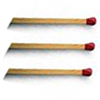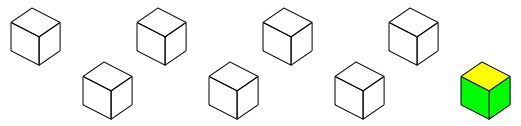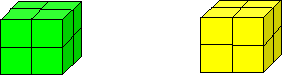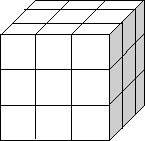#### You may also like### Construct-o-straws

Make a cube out of straws and have a go at this practical challenge.### Matchsticks

Reasoning about the number of matches needed to build squares that share their sides.### Little Boxes

How many different cuboids can you make when you use four CDs or DVDs? How about using five, then six?

# Green Cube, Yellow Cube

##### Age 7 to 11Challenge Level

There are eight small cubes. Each face of each cube is to be painted either green or yellow but each cube must use each of the two colours somehow.Work out how to paint the faces so that the cubes can be put together to make a $2$ by $2$ by $2$ cube that is green all over AND can be rearranged to make a $2$ by $2$ by $2$ cube that is yellow all over.Now work out how to paint the faces to make a $3$ by $3$ by $3$ green cube and a $3$ by $3$ by $3$ yellow cube.